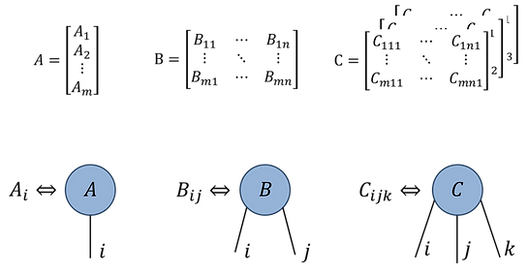top of page

Introduction

On this page we provide a brief introduction to some important concepts regarding tensor networks and their applications. There also exists many excellent introductory manuscripts and lectures, as suggested in the Links, where one could find a more comprehensive overview of these topics.

What are Tensor Networks used for?

Fundamentally, tensor networks serve to represent sets of correlated data, the nature of which can depend on the application in question. Some common applications include:

• the study quantum many-body systems, where tensor networks are used to encode the coefficients of a state wavefunction.

• the study classical many-body systems, where tensor networks are used to encode statistical ensembles of microstates (i.e. the partition function).

• big data analytics, where tensor networks can represent multi-dimensional data arising in diverse branches such as signal processing, neuroscience, bio-metrics, pattern recognition etc.

What are Tensor Networks used for?

Fundamentally, tensor networks serve to represent sets of correlated data, the nature of which can depend on the application in question. Some common applications include:

• the study quantum many-body systems, where tensor networks are used to encode the coefficients of a state wavefunction.

• the study classical many-body systems, where tensor networks are used to encode statistical ensembles of microstates (i.e. the partition function).

• big data analytics, where tensor networks can represent multi-dimensional data arising in diverse branches such as signal processing, neuroscience, bio-metrics, pattern recognition etc.

Why Tensor Networks?

Tensor network representations are useful for a number of reasons:

• tensor networks may offer a greatly compressed representation of a large structured data set (sometimes called "super" compression, since the ratio between the size of the compressed/uncompressed data can be staggeringly large!).

• tensor networks potentially allow for a better characterization of structure within a data set, particularly in terms of the correlations. In addition, the diagrammatic notation used to represent networks can provide a visually clear and intuitive understanding of this structure.

• tensor networks naturally offer a distributed representation of a data set, such that many manipulations can be performed in parallel.

• tensor networks allow a unified framework for manipulating large data sets. Common tasks, such as evaluating statistical information, are performed using a small collection of tensor network tools, which can be employed without any specific knowledge about the underlying data set or what it represents.

• tensor networks are often well suited for operating with noisy or missing data, as the decompositions on which they are based are typically robust.

##### The Fundamentals of Tensor Networks

For our purposes, a tensor can simply be understood as a multi-dimensional array of numbers. Typically we use a diagrammatic notation for tensors, where each tensor is drawn as a solid shape with a number of 'legs' corresponding to its order:• ###### order-3 tensorWe can form networks comprised of multiple tensors, where and index shared by two tensors denotes a contraction (or summation) over this index:

Notice that the example above on the left is equivalent to a matrix multiplication between matrices A and B, while the example on the right produces a rank-3 tensor D via the contraction of a network with three tensors. Even in this relatively the simple example, we see that the diagrammatic notation is already easier to interpret than the corresponding index equation!

In many applications, the goal is to approximate a single high-order tensor as a tensor network composed of many low-order tensors. Since the total dimension of a tensor (i.e. the parameters it contains) grows exponentially with its order, the latter representation can be vastly more efficient!

###### contains ~poly(N) parametersMuch of tensor network theory focuses on understanding how these representations work, and in what circumstances are expected to work. In contrast, tensor network algorithms typically focus on methods to efficiently obtain, manipulate, and extract information from these representations.

##### Tensor networks for Quantum Many-body Systems

One of the most common uses for tensor networks are representing quantum wavefunctions, which characterize the state of a quantum system.

Let us consider a d-level quantum system: a system where the state space is a d-dimensional (complex) vector space spanned by d orthogonal basis vectors. A (pure) state |ϕ〉 of the system is represented as a vector in this space, which can be specified as a superposition (or linear combination) of basis vectors according to a set of d complex amplitudes CiNow let us consider a quantum many-body system formed from a composition of N individual systems of dimension d. This could model, for instance, a condensed matter system such as a lattice of atoms, where each sub-system represents the state of an individual atom or electron. A pure state wavefunction |Ψ〉 of the composite system is given by specifying a complex amplitude for all possible configurations, of which there are d^N. This set of complex amplitudes is naturally represented as an order-N tensor C:In a typical many-body problem, we begin with a Hamiltonian which describes the how the subsystems interact with each other in the model under consideration, and the goal is to find the lowest energy eigenstate (or ground state) of the model. In this setting, tensor networks are most commonly used as ansatz (or assumed form) for quantum states, where the tensor network is used to (approximately) represent the tensor C of complex amplitudes. Common tensor network ansatz include MPS, TTN, MERA and PEPS.###### State Coefficient Tensor

The choice of best tensor network ansatz for a particular problem may depend on the geometry of the problem as well its physical properties. After choosing a specific tensor network ansatz to use, one typically begins by randomly initializing an instance of this network. Then, over many iterations, the parameters contained within the tensors are varied such as that the tensor network ansatz becomes a better approximation to the ground state of the quantum system under consideration. Common methods to do this parameter variation include energy minimization and Euclidean time evolution

An obvious question arises at this point: how to we know which many-body systems have ground states that can be well approximated by different tensor network ansatz? Fortunately the answer to this question is relatively well understood in terms of entangle scaling in quantum ground states in relation to the geometry of tensor network ansatz.

bottom of page